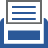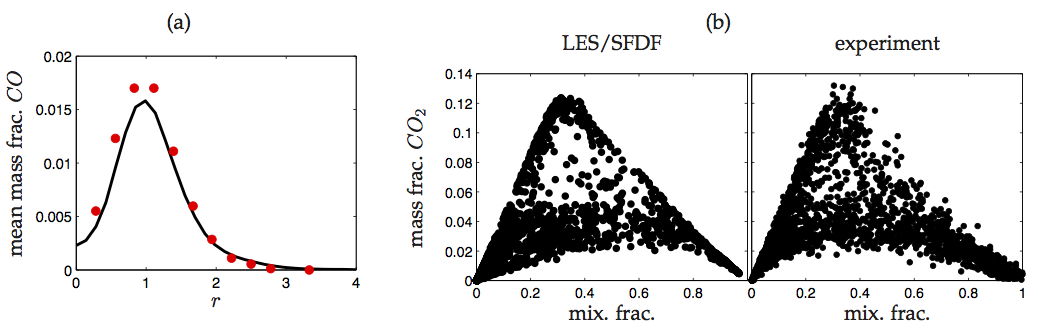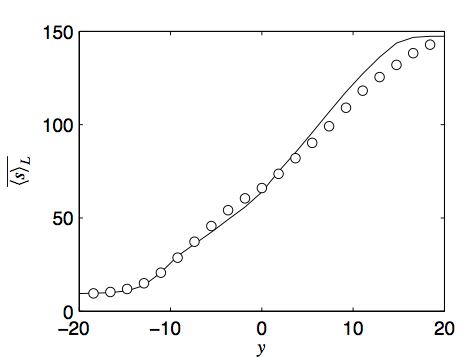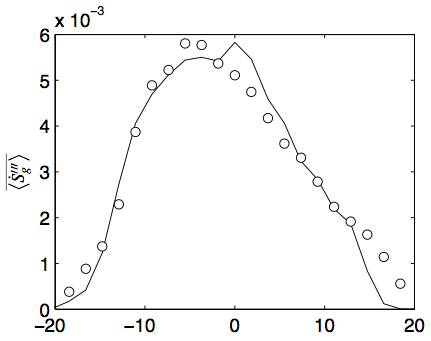##printer friendly Reports: DNI950912-DNI9: Large-Scale Simulation of Turbulent Flames with Detailed Chemistry

Reza Sheikhi, PhD, Northeastern University

High-fidelity simulation of turbulent combustion has become an important area of research, due to its enormous potential to help improve the performance and efficiency of combustion systems. The main objectives of this research are to develop and to apply a novel methodology for predictive modeling and simulation of complex turbulent reacting flows, and to advance the fundamental understanding of such flows. One of the most challenging issues in analysis of practical combustion systems is associated with the physics of turbulence. It is commonly accepted that the optimal means of capturing the detailed, unsteady physics of turbulent reacting flows is via large eddy simulation (LES). A challenging issue associated with LES is accurate modeling of the subgrid scale (SGS) quantities. The filtered-density function (FDF) methodology has proven particularly effective for this closure. The effectiveness of FDF is due to its inherent capability to account for the effects of chemical reaction in an exact manner. However, consideration of complex chemistry along with FDF is still a challenge, due to high computational costs. Therefore, the central part of this research is to develop and implement efficient methods to handle complex chemical kinetics in FDF simulations. In this period, significant progress has been made to achieve the objectives of the project. This enables application of FDF to investigate increasingly more complex turbulent flames. Alongside research activities, two Ph.D. students are being trained in this project who will continue contributing to the area beyond the duration of this grant. A summary of the progress made during this period is provided below.

1) Efficient implementation of detailed chemical kinetics:

Efficient methods are developed and implemented for tractable simulation of turbulent reacting flows using LES/FDF with complex chemistry. These include efficient stiff ordinary differential equation (ODE) solvers for direct integration of complex kinetics and the Rate-Controlled Constrained-Equilibrium (RCCE) method. The former is based on recent advances in mathematics of stiff ODEs. A solver is developed to integrate the chemical source term using Rosenbrock method. Matrix operations are handled by parallel sparse linear algebra routines. In RCCE, a reduced number of scalar equations are considered, governing the evolution of "constraints" on chemical reaction. Implementation of RCCE into FDF is conducted by considering a system of reactions for the constraints. Coupling of the RCCE method with the FDF has been accomplished. However, work is needed to ensure its computational efficiency and scalable parallelization on modern computing platforms.

2) Improvement of the FDF solvers for massively parallel platforms:

The objective of this task is to develop improved methodologies for efficient implementation of FDF on massively parallel platforms. While the major thrust of this research is on physical modeling of turbulent reacting flows, an issue intimately related to (any) LES is its actual computational feasibility. The FDF solution procedure involves very large number of Monte Carlo (MC) particles. With the large number of particles, due to intensity of detailed chemistry computations, the solver requires enormous computation time. To handle such intensive simulations, scalable parallelization at the MC particle level and balanced load among CPUs are essential. These problems are effectively addressed in this project by using a hybrid domain decomposition/particle partitioning parallelism strategy. In this approach, the computational domain is decomposed into sub-domains with (approximately) the same number of particles. In each sub-domain, particles are randomly and equally partitioned, and assigned to a pool of processors. The hybrid method has been implemented on distributed memory as well as shared-memory multi-processor architectures. The FDF is applied along with direct integration routine for LES of a non-premixed turbulent jet flame (Sandia flame F). Figure 1 shows some of the LES/FDF results. Mass fraction of CO predicted by the FDF, as shown in Fig. 1(a), is in reasonable agreement with the data. Figure 2(b) illustrates the flame structure, represented by the scatter plot of CO2 mass fraction against mixture fraction. As predictions demonstrate, all LES/FDF results compare reasonably well with the experimental data.

3) Development of the entropy FDF to study the efficiency losses in turbulent combustion:

Analyzing the data obtained from LES/FDF is necessary to improve the efficiency of processes involved in turbulent combustion. A new methodology is being developed that facilitates such analysis by including the transport of entropy within the FDF framework. The filtered entropy is considered in LES, which includes several unclosed source terms that contribute to entropy generation and hence, efficiency losses in combustion. These terms describe the entropy production due to viscous dissipation, heat conduction, mass diffusion and chemical reaction. All the unclosed terms in the filtered entropy transport equation are provided by the entropy FDF. An exact equation is developed for the FDF which is modeled by a set of stochastic differential equations (SDEs). The system of SDEs include a stochastic process for entropy and is solved by a Lagrangian MC procedure. The entropy FDF is applied to a turbulent shear layer. Results are validated by the data generated by direct numerical simulation (DNS) of the same layer. Figure 2, 3 show the comparison of filtered entropy and its production rate obtained from the entropy FDF and DNS.Figure 1. LES/FDF simulations of Sandia flame F using detailed chemistry: (a) radial variation of mean CO mass fraction, (b) the flame structure depicted by CO2 mass fraction scatter data. The solid line denotes the FDF predictions. The red circles denote the experimental data.Figure 2. Cross stream variation of Reynolds-averaged filtered entropy in turbulent temporal mixing layer simulations. The line denotes the entropy FDF results. The circles show DNS data.Figure 3. Cross stream variation of Reynolds-averaged filtered entropy production in turbulent temporal mixing layer simulations. The line denotes the entropy FDF results. The circles show DNS data.

ND
Solid Flow
Instabilities
Dr. Hrenya
UNI
Ionic Liquid
Cage Effects